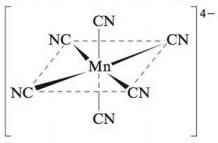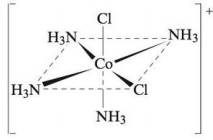# Name the following complex ions: a. b. c.### Chemistry: An Atoms First Approach

2nd Edition
Steven S. Zumdahl + 1 other
Publisher: Cengage Learning
ISBN: 9781305079243

#### Solutions

Chapter
Section### Chemistry: An Atoms First Approach

2nd Edition
Steven S. Zumdahl + 1 other
Publisher: Cengage Learning
ISBN: 9781305079243
Chapter 20, Problem 33E
Textbook Problem
1 views

## Name the following complex ions:a.b.c.(a)

Interpretation Introduction

Interpretation: The name of the given structures of complex ion is to be stated.

Concept introduction: Rules followed in the naming of coordination compound:

• In naming of coordination compound, the name of anion (ligand) comes first. Metal ion is named second, which is the name of element.
• An ‘o’ is added to the root name of anion.
• Greek prefix is used to express the number of ligands.
• Roman numeral is used to denote the oxidation state of metal ion.
• In case of more than one type of ligand present in a compound, they are named alphabetically.
• The suffix ‘-ate’ is added to metal ion if complex ion contains negative charge. Latin names are also used to identify the name of metals.

To determine: The name of the given structure of complex ion.

### Explanation of Solution

The given structure is,

Figure 1

The complex ion is [Mn(CN)6]4 .

The given structure contains negative charge.

Oxidation state of Cyanide (CN) ligand is 1 .

It is assumed that oxidation state of Manganese (Mn) is x .

The given complex compound contains six Cyanide ions and one Manganese ion

(b)

Interpretation Introduction

Interpretation: The name of the given structures of complex ion is to be stated.

Concept introduction: Rules followed in the naming of coordination compound:

• In naming of coordination compound, the name of anion (ligand) comes first. Metal ion is named second, which is the name of element.
• An ‘o’ is added to the root name of anion.
• Greek prefix is used to express the number of ligands.
• Roman numeral is used to denote the oxidation state of metal ion.
• In case of more than one type of ligand present in a compound, they are named alphabetically.
• The suffix ‘-ate’ is added to metal ion if complex ion contains negative charge. Latin names are also used to identify the name of metals.

To determine: The name of the given structure of complex ion.

(c)

Interpretation Introduction

Interpretation: The name of the given structures of complex ion is to be stated.

Concept introduction: Rules followed in the naming of coordination compound:

• In naming of coordination compound, the name of anion (ligand) comes first. Metal ion is named second, which is the name of element.
• An ‘o’ is added to the root name of anion.
• Greek prefix is used to express the number of ligands.
• Roman numeral is used to denote the oxidation state of metal ion.
• In case of more than one type of ligand present in a compound, they are named alphabetically.
• The suffix ‘-ate’ is added to metal ion if complex ion contains negative charge. Latin names are also used to identify the name of metals.

To determine: The name of the given structure of complex ion.

The name of complex ion [Co(NH3)5Cl]+ is pentaamminechlorocobalt(II).

### Still sussing out bartleby?

Check out a sample textbook solution.

See a sample solution

#### The Solution to Your Study Problems

Bartleby provides explanations to thousands of textbook problems written by our experts, many with advanced degrees!

Get Started

Find more solutions based on key concepts
Generally speaking, vegetable and fish oils are rich in saturated fat. T F

Nutrition: Concepts and Controversies - Standalone book (MindTap Course List)

Why is it difficult to detect planets orbiting other stars?

Foundations of Astronomy (MindTap Course List)

When two tuning forks are sounded at the same time, a beat frequency of 5 Hz occurs. If one of the tuning forks...

Physics for Scientists and Engineers, Technology Update (No access codes included)